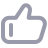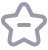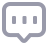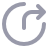# SQL为王：oracle标量子查询和表连接改写

479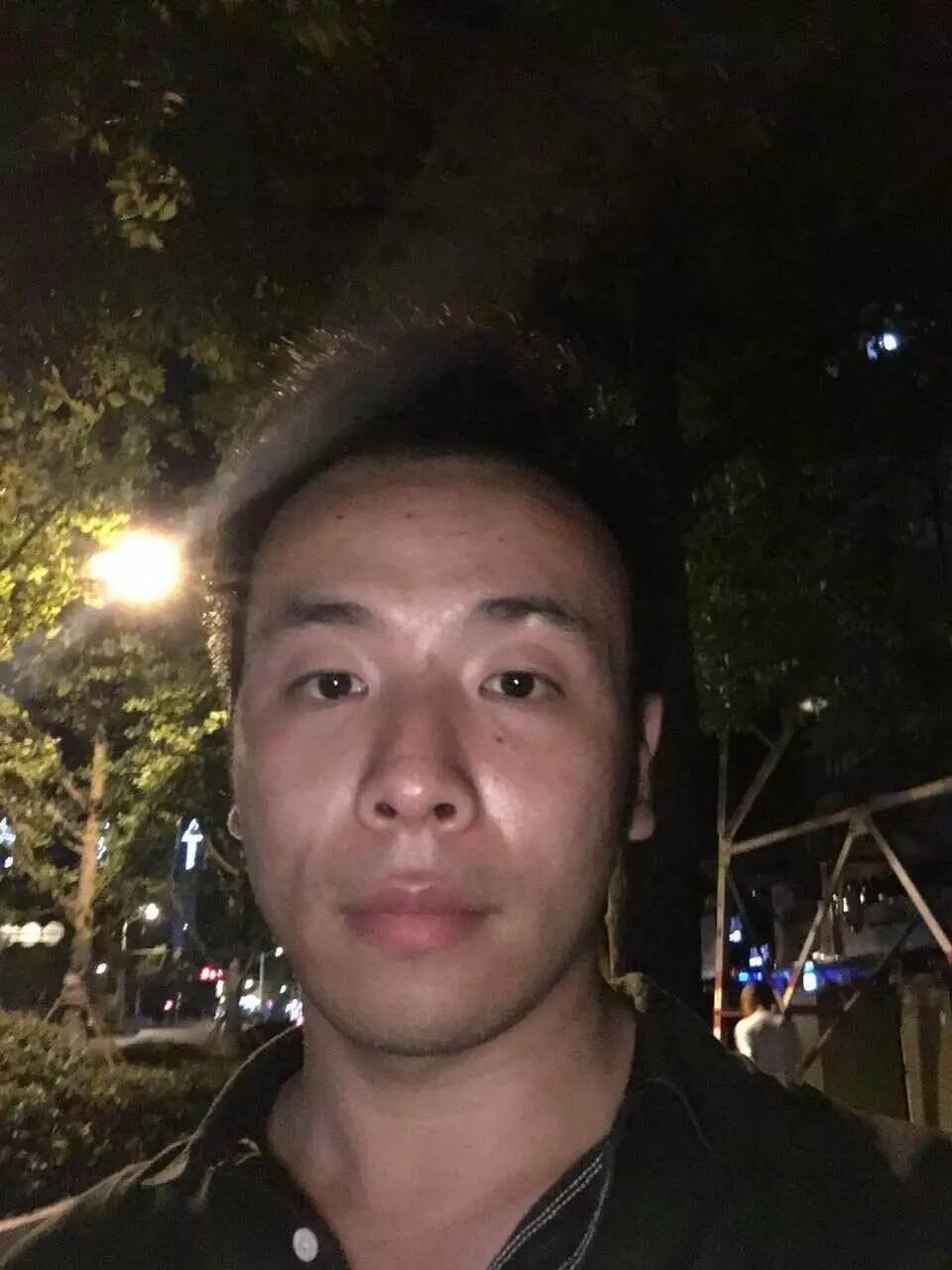Oracle允许在select子句中包含单行子查询，这个也就是oracle的标量子查询，标量子查询有点类似于外连接，当使用到外连接时我们可以灵活的将其转化为标量子查询。我们来看下面的例子：

SQL> create table t1 as select * from all_users;
Table created.
SQL> create table t2 as select * from all_objects;
Table created.
SQL> select a.object_id,(select b.username from t1 b where a.owner=b.username) from t2 a;

49812 rows selected.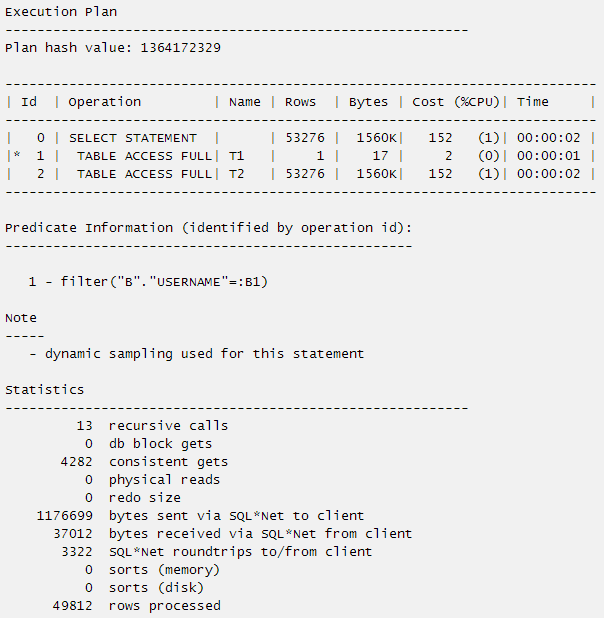49812 rows selected.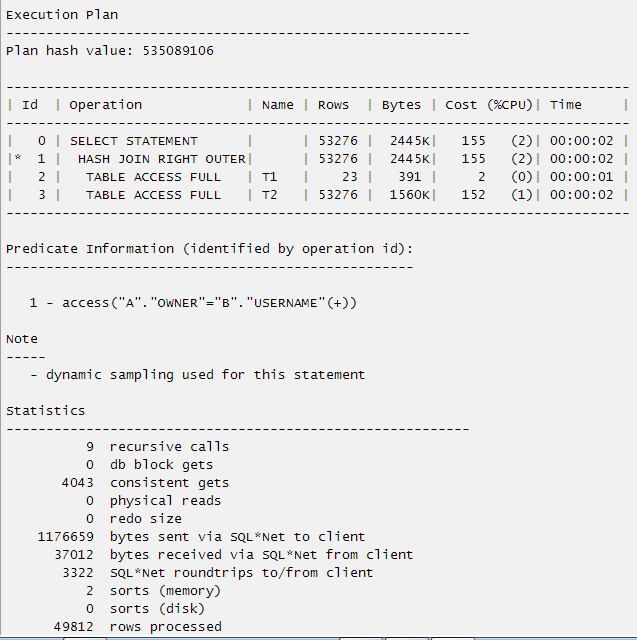29742 rows selected.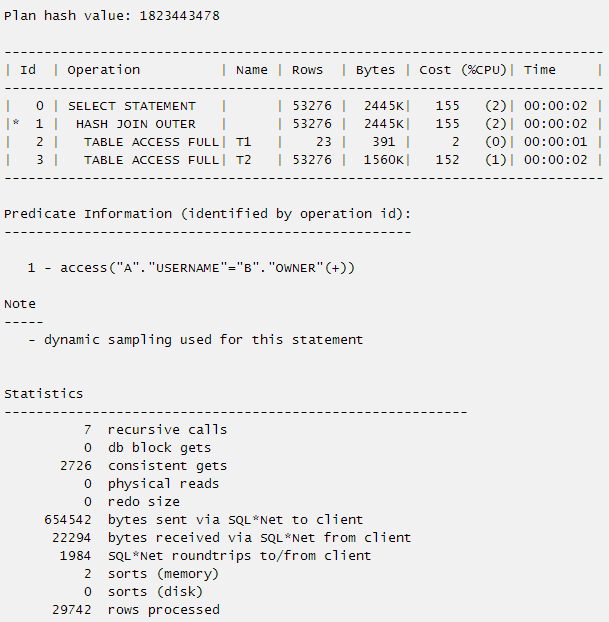SQL> select a.username,(select b.object_id from t2 b where a.username=b.owner) from t1 a;
*
ERROR at line 1:
ORA-01427: single-row subquery returns more than one row

SQL> select a.username,(select max(b.object_id) from t2 b where b.owner=a.username) from t1 a;
23 rows selected.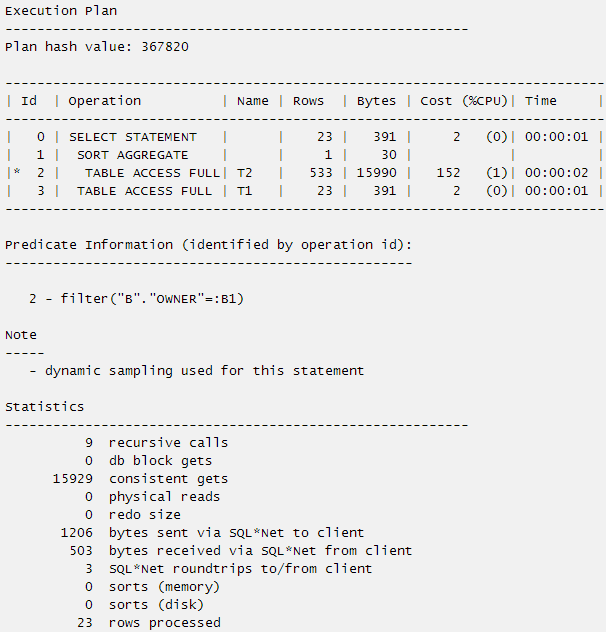1. 最简单的标量子查询

table :a(a1,a2),b(a1,b2)
select a2,(select b2 from b where b.a1=a.a1) from a

select a2,b2 from a,b where a.a1=b.a1(+);

2. 标量子查询带有聚合函数

table :a(a1,a2),b(a1,b2)
select a2,(select sum(b2) from b where b.a1 = a.a1) from a

SELECT a2, x.sum_value
FROM a,
( SELECT SUM (b2) sum_value, a1
FROM b
GROUP BY a1) x
WHERE a.a1 = x.a1(+);

3. 包含行转列的标量子查询改写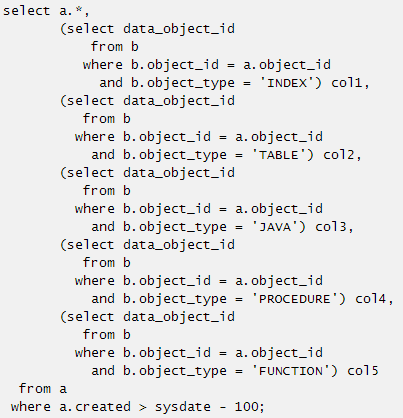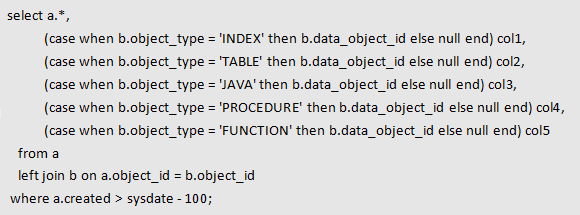4. 标量子查询同时包含行转列和聚合函数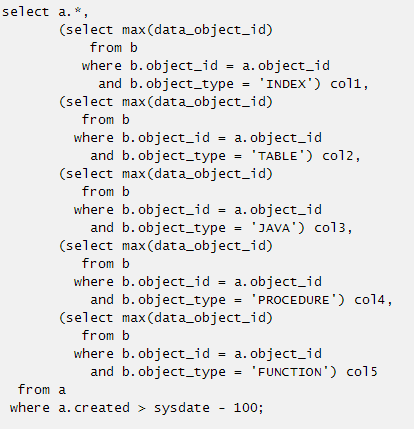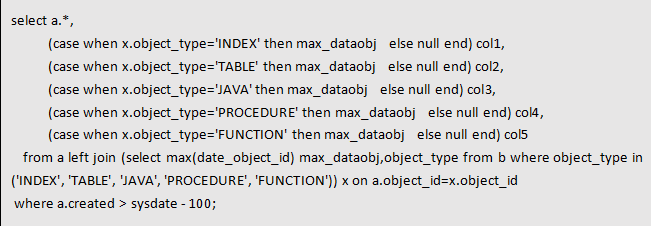5. 标量子查询中出现rownum=1或者rownum<2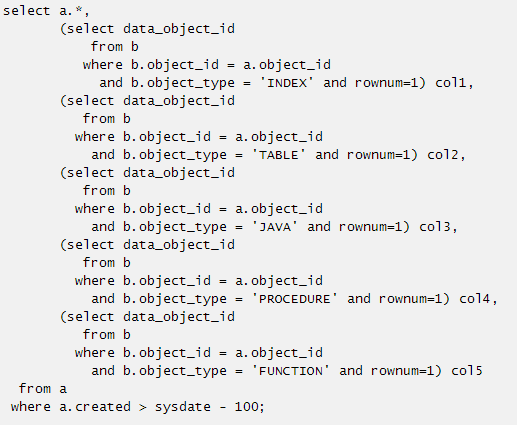select data_object_id
from b
where b.object_id = a.object_id
and b.object_type = 'INDEX' and rownum=1

select max(data_object_id)
from b
where b.object_id = a.object_id
and b.object_type = 'INDEX'
or
select min(data_object_id)
from b
where b.object_id = a.object_id
and b.object_type = 'INDEX'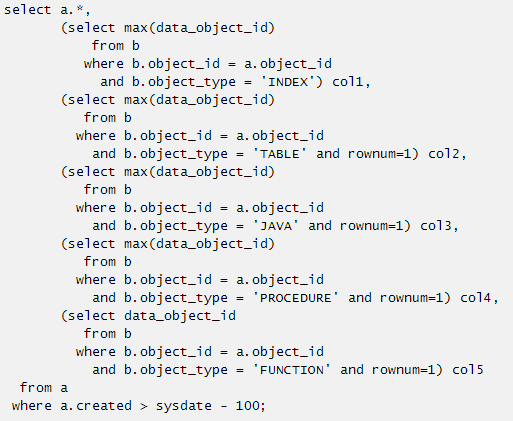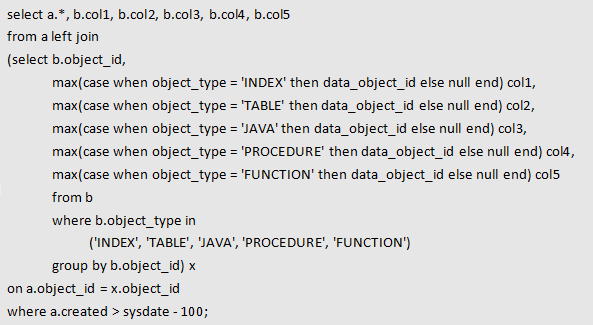6. 关于标量子查询改写后逻辑校验：

select a.job,
a.deptno,
(select distinct dname from dept2 b where b.deptno=a.deptno) as dname
from emp a

select distinct a.job,a.deptno,b.dname
from emp a
left join dept2 b on b.deptno=a.deptno;

select a.job,a.deptno,b.dname。
from emp a
left join (select dname,deptno from dept2 group by dname,deptno)b
on b.deptno=a.deptno

7. 不等连接的标量子查询改写：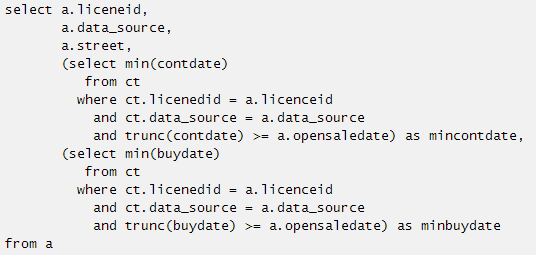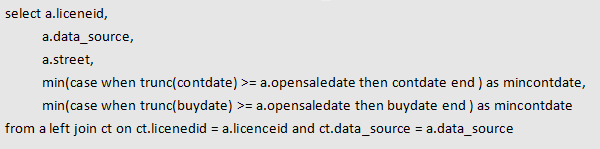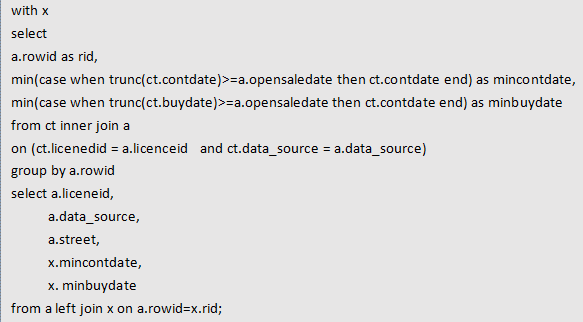ct.licenedid = a.licenceid、

and ct.data_source = a.data_source、

trunc(ct.contdate)>=a.opensaledate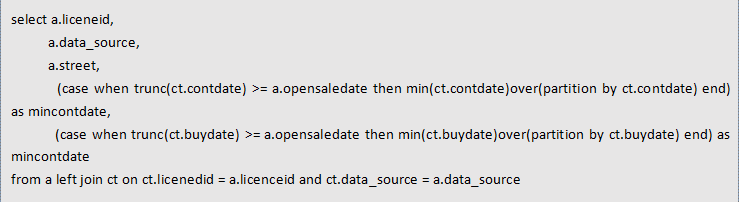------The end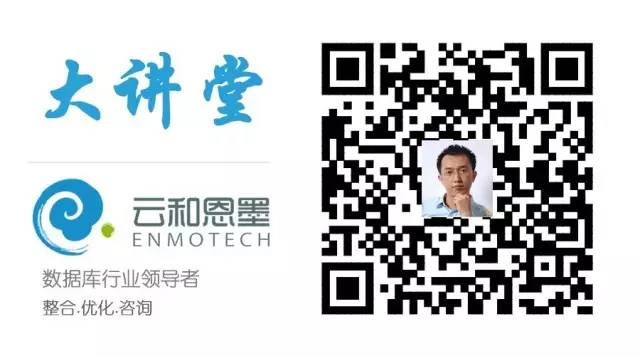## 深入剖析 ORA-04031 的前世今生

2016DTCC, 2016数据库大会PPT；

DBALife,"DBA的一天"精品海报大图；

12cArch,“Oracle 12c体系结构”精品海报；

DBA01，《Oracle DBA手记》第一本下载；

YunHe“云和恩墨大讲堂”案例文档下载；